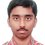# Circular Motion Problem

A rod of length L is pivoted at one end & rotated with a uniform angular velocity in a horizontal plane. Let $T_{1}$ & $T_{2}$ be the tensions at points L/4 & 3L/4 respectively away from pivoted ends. Then

(a) $T_{1}$ > $T_{2}$

(b) $T_{1}$ = $T_{2}$

(c) $T_{1}$ < $T_{2}$

(d) Relation between $T_{1}$ & $T_{2}$ depends on whether the rod rotates clockwise or anticlockwiseNote by Nishant Sharma
6 years, 3 months ago

MarkdownAppears as
*italics* or _italics_ italics
**bold** or __bold__ bold
- bulleted- list
• bulleted
• list
1. numbered2. list
1. numbered
2. list
Note: you must add a full line of space before and after lists for them to show up correctly
paragraph 1paragraph 2

paragraph 1

paragraph 2

[example link](https://brilliant.org)example link
> This is a quote
This is a quote
    # I indented these lines
# 4 spaces, and now they show
# up as a code block.

print "hello world"
# I indented these lines
# 4 spaces, and now they show
# up as a code block.

print "hello world"
MathAppears as
Remember to wrap math in $ ... $ or $ ... $ to ensure proper formatting.
2 \times 3 $2 \times 3$
2^{34} $2^{34}$
a_{i-1} $a_{i-1}$
\frac{2}{3} $\frac{2}{3}$
\sqrt{2} $\sqrt{2}$
\sum_{i=1}^3 $\sum_{i=1}^3$
\sin \theta $\sin \theta$
\boxed{123} $\boxed{123}$

## Comments

Sort by:

Top Newest

The tension at L/4 $=\frac{3}{4}M\omega^2[\frac{1}{2}\frac{3}{4}L]$. The tension at 3L/4 $=\frac{1}{4}M\omega^2[\frac{1}{2}\frac{1}{4}L]$. Therefore $T_1 > T_2$.

- 6 years, 3 months ago

Log in to reply

I 'll go with (a) :D

- 6 years, 3 months ago

Log in to reply

Hey, please show me the thinking or the concept.

- 6 years, 3 months ago

Log in to reply

Tension must acount for the centripital acceleration for both the cases. That's all I used with a bit of calculation to compute the centripital acceleration and mass. Am I correct?

- 6 years, 3 months ago

Log in to reply

i think your concept of creating tension in the rod becuase of centripetal accelaration is right and i think this is the key of this problem and we don't have to take it into consideration whether the rod is rotating clockwise or anticlockwise because for both of the two cases as the rod is rotating in horizontal plane would create same centripetal accleration at same distance........please let me know if furthermore anything should be thought of....

- 6 years, 3 months ago

Log in to reply

$a$ is correct...:)

- 6 years, 3 months ago

Log in to reply

C) t1 <t2

- 4 years, 9 months ago

Log in to reply

http://www.askiitians.com/forums/Mechanics/10/5212/circular-motion.htm

- 1 year, 12 months ago

Log in to reply

Consider the portion of the rod between L/4 and 3L/4. T1 acts on that portion in a radially inward direction. T2 acts on that portion in a radially outward direction. The net force on that portion must be radially inward. Therefore, T1 > T2.

- 6 years, 3 months ago

Log in to reply

You may be correct. But how far I know tension in a segment of rod always acts towards the mid-point of that section. Anyways can you give me a mathematical solution to this problem ? Including the free-body diagram ?(if possible)

- 6 years, 3 months ago

Log in to reply

×

Problem Loading...

Note Loading...

Set Loading...